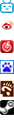### 强迫症带来的矛盾```\$lefth=0;
\$righth=30;
\$left=Array();
\$right=Array();
foreach (\$arr as \$time=>\$posts){
\$hei=64+26*(count(\$posts));
if (\$lefth<=\$righth){
if (\$lefth+30>\$righth){
\$right[]=\$lefth+30-\$righth;
\$righth=\$lefth+30;
}
\$left[\$time]=\$posts;
\$lefth+=\$hei;
}else{
if (\$righth+30>\$lefth){
\$left[]=\$righth+30-\$lefth;
\$lefth=\$righth+30;
}
\$right[\$time]=\$posts;
\$righth+=\$hei;
}
}```

2016
04 10

Older

0

1766